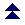Index

# Vector variables and operations

This page contains operations and conditional statements involving Vector variables, introduced in version 3.416. All vectors are 3-dimensional, containing an x, y and z value. Relative to the AW coordinate system, x is WE (west is positive), y is Altitude (up is positive), z is NS (north is positive), expressed in metres.

 /v_vecR = /v_vec2 /v_vecR = @a /v_vecR = &a /v_vecR = ~a Direct assignment of a vector or position (location, person, object) to Vector variable /v_vecR. Note: data transmission between location, person, object variables and vector variables is done in metres. All vectors implying distance or translations must have its values in metres. VecSet /v_vecR /v_vec2 VecSet /v_vecR @a VecSet /v_vecR &a VecSet /v_vecR ~a VecSet /v_vecR %x %y %z VecSet /v_vecR \$a Assignement to Vector variable /v_vecR. The first four are similar to the direct assignment. The last two require 3 numeric elements. They can be contained in Numeric variables VecSet /v_vecR %x %y %z or written literally as VecSet /v_vecR 3.0 5.3 -1.95 or in a String variable \$a = "3.0 5.3 -1.95" VecSet /v_vecR \$a Note: data transmission between location, person, object variables and vector variables is done in metres. All vectors implying distance or translations must have its values in metres. VecGet @a /v_vecR VecGet &a /v_vecR VecGet ~a /v_vecR VecGet %x %y %z /v_vecR VecGet \$a /v_vecR Assignement from Vector variable /v_vecR to location, person or object variables @a, &a, ~a, or to the Numeric variables %x %y %z, or to a string variable. Note: data transmission between location, person, object variables and vector variables is done in metres. All vectors implying distance or translations must have its values in metres. VecLength %L /v_vec Stores the length of the vectore in %L. The length of a vector is the square root of the sum of the squares of its components (i.e. the square root of the norm). VecNorm %n /v_vec Stores the norm of the vectore in %n. The norm of a vector is the sum of the squares of its components. VecAdd /vecR /vec1 /vec2 Corresponding elements of /v_vec1 and /v_vec2 are added, and the result is stored in /v_vecR. VecSbt /vecR /vec1 /vec2 Corresponding elements of /v_vec2 are subtracted from /v_vec1, and the result is stored in /v_vecR, or in ordinary maths: vecR = vec1 - vec2. VecScale /v_vecR /v_vec1 %s Each element of /v_vec1 is mutiplied by %s, and the result is stored in /v_vecR. VecCombine /v_vecR /v_vec1 /v_vec2 %s1 %s2 /v_vec1 is scaled by %s1, /v_vec2 is scaled by %s2, the scaled vectors get added and the result is stored in /v_vecR. VecDot %r /v_vec1 /v_vec2 Calculates the dot product of two vectors and stores the result in Numeric variable %r (dot product is a scalar). VecCross /v_vecR /v_vec1 /v_vec2 Calculates the cross product of two vectors, and stores the result in vector /v_vecR. VecLerp /v_vecR /v_vec1 /v_vec2 %f Calculates the linear interpolaton between two vectors for a factor %f (%f must be between 0.0 and 1.0), and stores the result in /v_vecR. VecNormalise /v_vec VecNormalize /v_vec Changes the length of the vector to 1.0. To do so, it calculates the length of the vector, and then divides each elemnt by the length. If all elements = 0.0, the division can not take place, and the vector will be set to (0, 0, 1). VecTransform /v_vecR /v_vec /m_mat VecTransform /v_vecR /m_mat Applies matrix /m_mat to vector /v_vec and stores the transformed vector in /v_vecR. A shorter version transforms /v_vecR directly.

An example script using vectors and matrices to build a circle of objects can be found here.Index